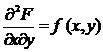# Joint Probability Distribution Function Assignment Help

let (X, Y) be a two dimensional random variable then their joint distribution function is denoted by FXY (X,Y) and it represents the probability that simultaneously the observation (X,Y) will have the property (X ≤ x and Y ≤ y) that isFXY (X,Y) = P( -∞ <X ≤ x, -∞ < Y ≤ y)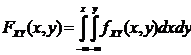Where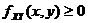And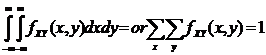Properties of joint distribution function:

1. 1. for the real numbers a1, b1, a2 and b2

## Joint Probability Distribution Function Assignment Help By Online Tutoring and Guided Sessions at AssignmentHelp.Net

P(a1 < X ≤ b1, a2 < X ≤ b2) = FXY (b1, b2) + FXY (a1, a2) - FXY (a1, b2) - FXY (b1, a2)

1. 2. F (-∞, y) = 0 = F(x, +∞), F(-∞,+∞) =1
2. 3. If the density function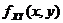is continuous at (x,y) then